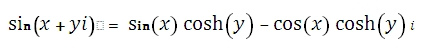# SQL Server IMSIN function

Updated: 27 August 2010

Use IMSIN to return the sine of a complex number in x + yi or x + yj text format. The sine of a complex number is computed by:Syntax
SELECT [wctEngineering].[wct].[IMSIN] (
<@inumber, nvarchar(4000),>)
Arguments
@inumber
is the value at which to evaluate the function. @inumber is an expression of type varchar or of a type that can be implicitly converted to varchar and must be in the format x + yi or x + yj, where x is a real number that can be converted to float and yi (or yj) is an imaginary number, where the y part can be converted to float.
Return Types
varchar(4000)
Remarks
·         Use COMPLEX to convert real and imaginary coefficients into a complex number
Examples

select
wct.IMSIN('7+24i')

This produces the following result
-----------------------
8701499125.56623+9985104444.63206i

(1 row(s) affected)

### SupportCopyright 2008-2021 Westclintech LLC         Privacy Policy        Terms of Service